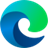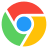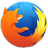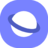### Description

The CSS `linear-gradient()` function creates a linear gradient, which is a smooth transition between two or more colors along a straight line. Gradients can be used to create a variety of visual effects, such as adding depth, dimension, and movement to web pages.

To use the `linear-gradient()` function, you must define at least two color stops. Color stops are the colors that you want to blend together in the gradient. You can also define a starting point and a direction for the gradient.

The syntax for the `linear-gradient()` function is as follows:

``````linear-gradient(direction, color-stop1, color-stop2, ...);
``````
• `direction`: The direction of the gradient. Can be one of the following values: `to top`, `to bottom`, `to left`, `to right`, or an angle in degrees.
• `color-stop`: A color stop, which is a color value followed by an optional stop position. The stop position can be a percentage between 0% and 100%, or a length along the gradient axis.

If you do not define a starting point or direction for the gradient, it will start in the top-left corner of the element and run to the bottom-right corner.

Here is an example of a simple linear gradient:

``````div {
}
``````

This will create a gradient background that transitions from red on the left to blue on the right.

You can also use the `linear-gradient()` function to create more complex gradients, such as gradients with multiple colors or gradients with different directions.

Here is an example of a more complex linear gradient:

``````div {
background: linear-gradient(45deg, red, 50%, green, 100% blue);
}
``````

This will create a gradient background that transitions from red on the top-left to green on the bottom-right, with a stop in the middle at 50% where the color is blue.

Linear gradients can be used to create a variety of visual effects on web pages. For example, you can use them to create:

• Colorful backgrounds
• And much more!

Linear gradients are a powerful and versatile CSS tool that can be used to add visual interest and style to your web pages.

### Syntax

```linear-gradient(
[ <angle> | to <side-or-corner> ,]? <color-stop-list> )
---------------------------------/ ----------------------------/
Definition of the gradient line        List of color stops

where <side-or-corner> = [ left | right ] || [ top | bottom ]
and <color-stop-list> = [ <linear-color-stop> [, <color-hint>? ]? ]#, <linear-color-stop>
and <linear-color-stop> = <color> [ <color-stop-length> ]?
and <color-stop-length> = [ <percentage> | <length> ]{1,2}
and <color-hint> = [ <percentage> | <length> ]```

### Values

• <side-or-corner>The position of the gradient line's starting point. If specified, it consists of the word to and up to two keywords: one indicates the horizontal side (left or right), and the other the vertical side (top or bottom). The order of the side keywords does not matter. If unspecified, it defaults to 'to bottom'.
• <angle>A value that specifies the direction of the gradient. 0deg points upward, and positive angles represent clockwise rotation, so 90deg points toward the right.
• <linear-color-stop>A color-stop's <color> value, followed by one or two optional stop positions, (each being either a <percentage> or a <length> along the gradient's axis).
• <color-hint>The color-hint is an interpolation hint defining how the gradient progresses between adjacent color stops. The length defines at which point between two color stops the gradient color should reach the midpoint of the color transition. If omitted, the midpoint of the color transition is the midpoint between two color stops.

### Example

``<div class="gradient"> Example of the CSS Function Linear Gradient.</div>``
``````.gradient {
color: white;
The following table will show you the current browser support for the CSS `linear-gradient()` function.
 Desktop12 26 16 12.1 7
 Tablets / Mobile26 16 12.1 7 1.5 37